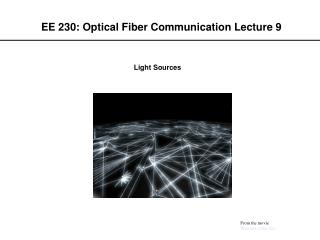Download PresentationEE 230: Optical Fiber Communication Lecture 9EE 230: Optical Fiber Communication Lecture 9 - PowerPoint PPT Presentation

Download PresentationEE 230: Optical Fiber Communication Lecture 9
An Image/Link below is provided (as is) to download presentation

Download Policy: Content on the Website is provided to you AS IS for your information and personal use and may not be sold / licensed / shared on other websites without getting consent from its author. While downloading, if for some reason you are not able to download a presentation, the publisher may have deleted the file from their server.

- - - - - - - - - - - - - - - - - - - - - - - - - - - E N D - - - - - - - - - - - - - - - - - - - - - - - - - - -
Presentation Transcript

1. EE 230: Optical Fiber Communication Lecture 9 Light Sources From the movie Warriors of the Net

2. Conditions for gain (lasing) • E2-E1<Fc-Fv (population inversion) • g(1/L)ln(1/R)+ (net gain) • =2nL/p, p an integer (phase coherence)

3. Reflectivity

4. Longitudinal mode spacing

5. Laser Diode Structure and Optical modes

6. Conditions for continuous lasing (steady state) • Net rate of change of density of conduction band electrons is zero (injection minus recombination and depletion) • Net rate of change of density of photons created is zero (stimulated emission minus leakage and spontaneous emission)

7. Laser Electrical Models Simple large signal model Package Lead Inductance Bond wire Inductance Laser contact resistance Use a large signal diode model for the laser junction, this neglects the optical resonance Package Lead Capacitance Laser Junction Laser Pad Capacitance More exactly the laser rate equations can be implemented in SPICE to give the correct transient behavior under large signal modulation Assume that the light output is proportional to the current through the laser junction Small signal model (Hitachi)

8. Steady-state lasing conditions

9. Turn-on delay

10. Input Current Ib=0 Output Light Signal Ib=0.5Ith Turn on Delay (ns) Ib=0.9Ith d Turn-on Delay To reduce the turn on delay: • Use a low threshold laser and make Ip large • Bias the laser at or above threshold

11. Relaxation oscillation Decays as e-t/2, where and with a freqency , where

12. Modulation frequency Difference between optical output at modulation frequency m and steady-state output is proportional to

13. Resonance Frequency Semiconductor lasers exhibit an inherent second order response due to energy “sloshing” back-and-forth between excited electrons and photons

14. Large Signal Transient Response

15. Effects of current and temperature • Applying a bias current has the same effect as applying a pump laser; electrons are promoted to conduction band. Fc and Fv get farther apart as well • Increasing the temperature creates a population distribution rather than a sharp cutoff near the Fermi levels

16. Fabry Perot Laser Characteristics (Hitachi Opto Data Book)

17. Quantum efficiency • Internal quantum efficiency i, photons emitted per recombination event, determined empirically to be 0.650.05 for diode lasers • External quantum efficiency e given by

18. Total quantum efficiency Equal to emitted optical power divided by applied electrical power, or he/qV For GaAs lasers, TQE  50% For InGaAsP lasers, TQE  20%

19. Chirping Current modulation causes both intensity and frequency modulation(chirp) As the electron density changes the gain (imaginary part of refractive index ni) and the real part of the refractive index (nr) both change. The susceptability of a laser to chirping is characterized by the alpha parameter. 1-3 is expected for only the very best lasers Chirping gets worse at high frequencies Relaxation oscillations will produce large dp/dt which leads to large chirping Damping of relaxation oscillations will reduce chirp Correctly adjusting the material composition and laser mode volume can reduce 

20. Reflection Sensitivity Problem Solution R. G. F. Baets, University of Ghent, Belgium

21. Example A GaInAs diode laser has the following properties: • Peak wavelength: 1.5337 m • Spacing between peaks: 1.787x10-3m • J/Jth=1.2 What are the turn-on delay time, the cavity length, the threshold electron density, and the threshold current?

22. Turn-on delay time =3.7 ln(1.2/1.2-1) = 6.63 ns

23. Cavity length L = (1.5337)2/(2)(3.56)(1.787x10-3) = 184.9 m

24. Threshold electron density R = 0.3152 g(1/L)ln(1/R)+ gth=1/.01849 ln(1/.3152)+100=162.4 cm-1 From figure, N=1.8x1018 cm-3

25. Threshold current J/2de = I/2deLW Ith=(0.5x10-4)(1.6x10-19)(1.8x1018)(.01849)(4x10-4)/(3.7x10-9) Ith=29 mA

26. Laser Diode Structures Most require multiple growth steps Thermal cycling is problematic for electronic devices

27. Laser Reliability and Aging

28. Power degradation over time Lifetime decreases with current density and junction temperature

29. Monitor Photodiode Laser - Data Vref + -5V Problems with Average Power Feedback control of Bias Problem: L-I curves shift with Temperature and aging Turn on delay increased Frequency response decreased Average Power Average Power Light Light Current Current L-I Characteristic with temperature dependent threshold Ideal L-I Characteristic Output power, frequency response decreased Light Average Power Current Average number of 1s and Os (the “Mark Density”) is linearly related to the average power. If this duty cycle changes then the bias point will shift L-I Characteristic with temperature dependent threshold and decreased quantum efficiency